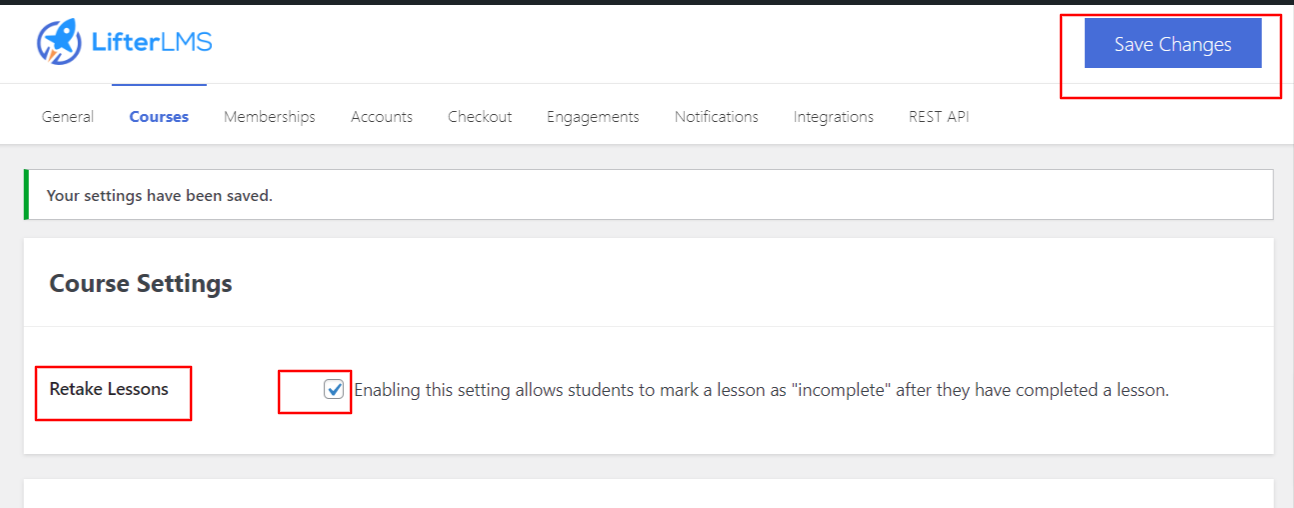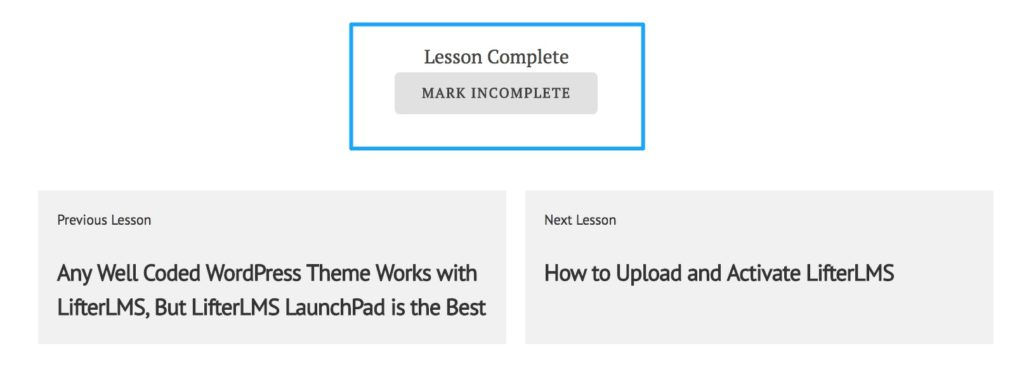# How can students retake lessons?

When a student completes a lesson, that lesson is marked as completed. Later, if the student wants to retake the lesson, the lesson remains complete.

Our Retake Lessons feature works as a solution to this problem. So that the student can easily mark the lesson as incomplete after completing a lesson.

To enable this feature, follow the below steps:

Step 1: Navigate to wp-admin > LifterLMS > Courses

Step 2: Enable the Retake Lessons option > Save Change.After a lesson has been completed, each student may now mark a completed lesson as Incomplete, allowing them to retake that lesson.If you don’t want to provide this ability to your students to mark a lesson as incomplete. Admins can do this process by themself. We have documentation on How Can I Mark a Lesson Complete or Incomplete for My Students?